# What is Terminal Value?

The Terminal Value (TV), or as it is also known, continuing value or horizon value, is the value of an investment or a business at the end of a specific period.

A firm or project potentially has an infinite life, meaning its value is the Net Present Value (NPV) of cash flows for an indefinite period. However, forecasting beyond a specified period becomes impractical and can introduce various risks, as it limits the validity of the model due to the high uncertainty added to the model assumptions.

Such cases are where we use Terminal Value.

It helps us estimate the value of a business beyond a specific forecast period.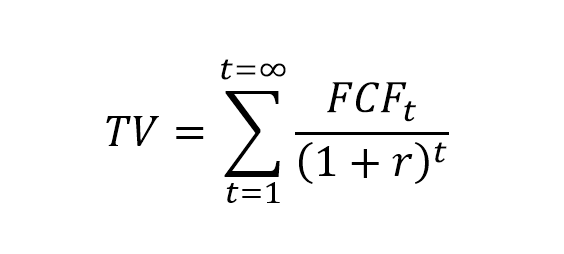Where:

• t is the period;
• FCF is the free cash flow; and
• r is the discount rate, often WACC (weighted average cost of capital).

The Terminal Value shows us the current present value of all future cash flows, after the point to which we can forecast. It is commonly used in DCF analysis, when calculating the value of a business. TV is the second part of the DCF model, the value after the forecast period.

There are two most common methods to calculate the Terminal Value, and also a third, less popular method:

• Perpetuity Growth Approach;
• Exit Multiple Approach; and
• No Growth perpetual approach.

We will look into the methods and see if one is preferred compared to the others.

## Perpetuity Growth Method

The Perpetuity Growth Method, also known as the Gordon Growth Model, is the preferred method for scholars and academics, as it is backed with a math theory.

It assumes that the company will continue to grow at a constant rate and the return on capital will exceed the cost of capital, and therefore we mostly apply it for mature companies that have achieved a stable growth.

The formula for this method starts from the first period FCF, after our forecast: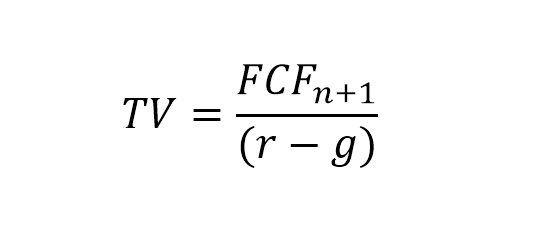Where:

• n is the last period in the forecast;
• g is the growth rate.

As we do not have this Free Cash Flow estimated, we can present the formula like this: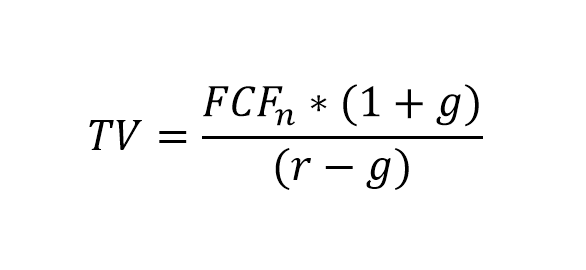For this approach, it is critical to determine the right growth rate, and analysts usually drop it to arrive at a more conservative terminal value.

## Exit Multiple Method

The assumption for this method is that we value the business via a multiple of some financial metrics (usually EBITDA or EBIT) based on currently observed comparable trading multiples for similar firms in the industry.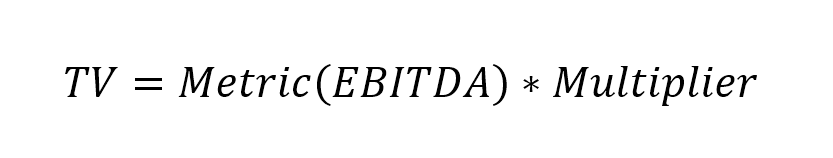Multiple of EBITDA measures the firm value of the enterprise, which is the value of business operations. This method only considers the operational value of the business. Although EBITDA is the most common financial statistic used, we can also use other metrics:

• EBIT;
• P/E multiple;
• Market to book;
• Price to Revenue.

## No Growth Perpetuity Method

This is not a popular approach, but we can use it in highly competitive industries where the opportunity to earn excess returns is close to zero. The assumption in this method is that the growth rate (g) is close to zero and therefore return on capital is equal to cost of capital.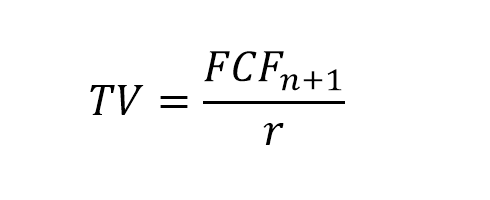# Which to use?

Industry professionals prefer the Exit Multiple approach as it compares the value of the business to something observable on the market.

On the other hand, academics prefer the Perpetuity Growth approach as it has more sound theory behind it.

Some practitioners take a hybrid approach and use an averaged combination of both methods.

# Considerations

Because Terminal Value is usually the more significant portion of Enterprise Value (EV), valuation models are susceptible to assumptions relating to the TV. We typically represent this via some sensitivity analysis.

It is also important to remember that after calculating TV, we do not include it directly in our EV calculation, but rather discount it to its present value.TV is critical to financial modeling for valuations, and a highly precise estimate of TV is crucial as it is often the more substantial portion of the total value.

# Example Calculation

To better understand Terminal Value, let us take a look at an example of how to calculate it.

We first create a forecast of the performance of the company for the next three periods, based on our historical data available for 2X16, 2X17, and 2X18.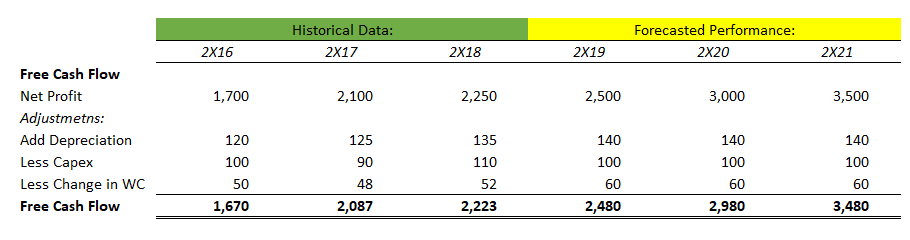We can set up our assumptions – a discount rate (r) of 10%, which is the weighted average cost of capital for the company, and an expected growth rate (g) after 2X21 at 4% in perpetuity.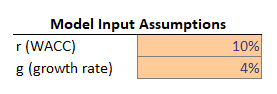We will start by calculating the net present value of the forecasted free cash flows for the three periods. It is easy to do with Excel’s npv() function. We will need this to calculate the Enterprise Value of the company later.We then calculate the Terminal Value of the company at the end of the third forecasted period, using the formula from the perpetual growth model. We also discount it to the end of the last historical period.We can then add the net present values of the forecasted period free cash flows and the terminal value, discounted to present value, to arrive at the enterprise value. We can then get to the equity value by adjusting for financial debt and cash. Dividing this over the number of shares of the company, we can calculate the value per share.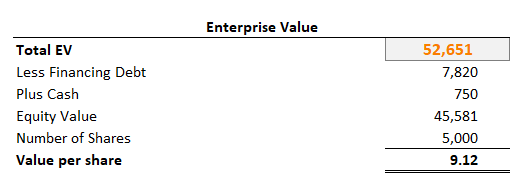# Conclusion

Terminal Value is an essential component in estimating the Discounted Cash Flow as it usually accounts for more than half of the total enterprise value. It is critical that we use the correct assumptions for the discount rate and the growth, or exit multiple, as even a 1% change in the assumptions can lead to significant deviations in the calculated enterprise value. However, Terminal Value provides us with the easiest way to calculate the value of the expected free cash flow of the company beyond the period of the explicit forecasted model.

Today we took a look at Terminal Value, what is the concept behind it and how to calculate it. Do not forget to download the Excel working file below:

## Get a FREE Excel Benchmark Analysis Template## FCCA, FMVA

Hi! I am a finance professional with 10+ years of experience in audit, controlling, reporting, financial analysis and modeling. I am excited to delve deep into specifics of various industries, where I can identify the best solutions for clients I work with.

In my spare time, I am into skiing, hiking and running. I am also active on Instagram and YouTube, where I try different ways to express my creative side.

The information and views set out in this publication are those of the author(s) and do not necessarily reflect the official opinion of Magnimetrics. Neither Magnimetrics nor any person acting on their behalf may be held responsible for the use which may be made of the information contained herein. The information in this article is for educational purposes only and should not be treated as professional advice. Magnimetrics and the author of this publication accept no responsibility for any damages or losses sustained in the result of using the information presented in the publication.